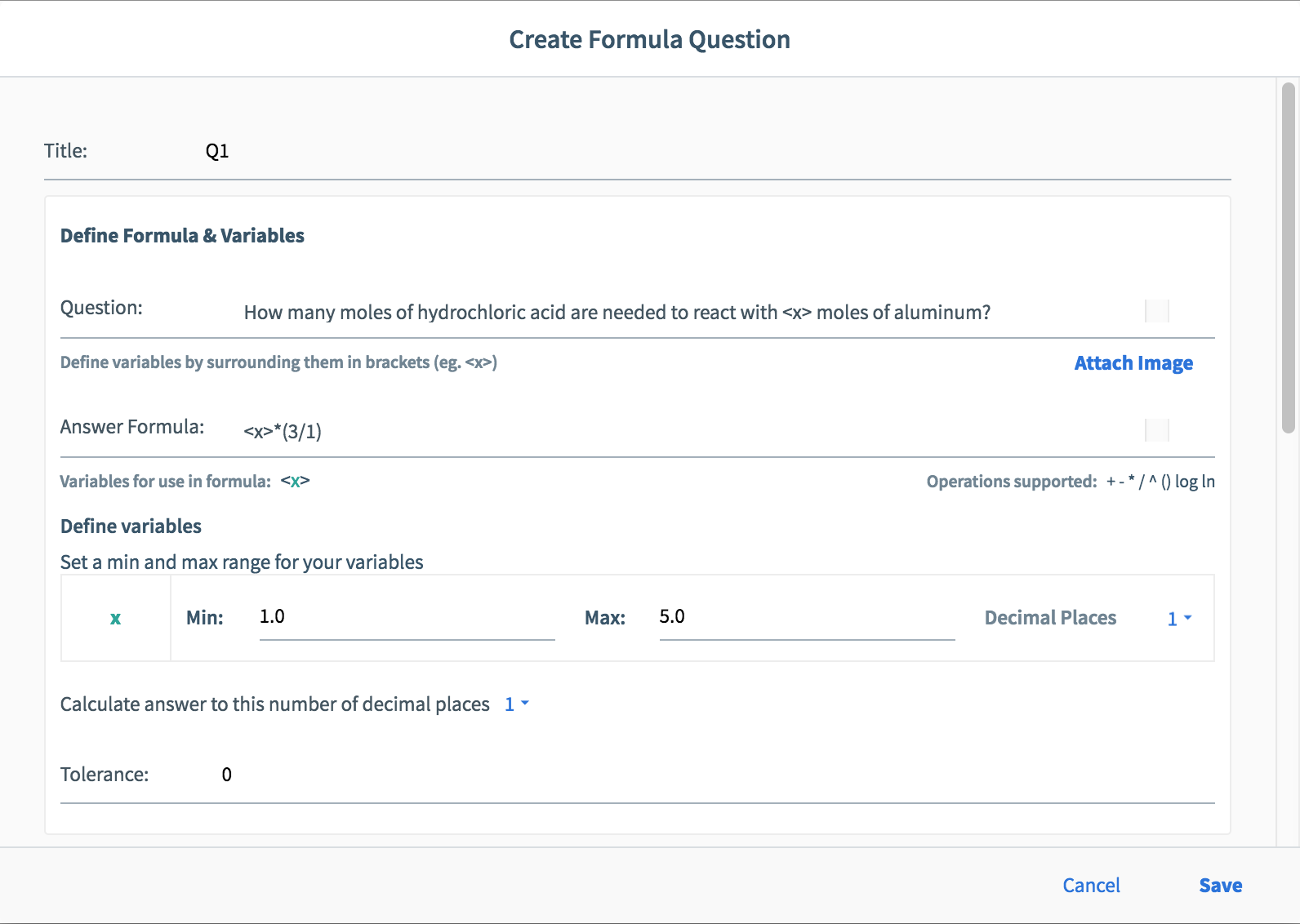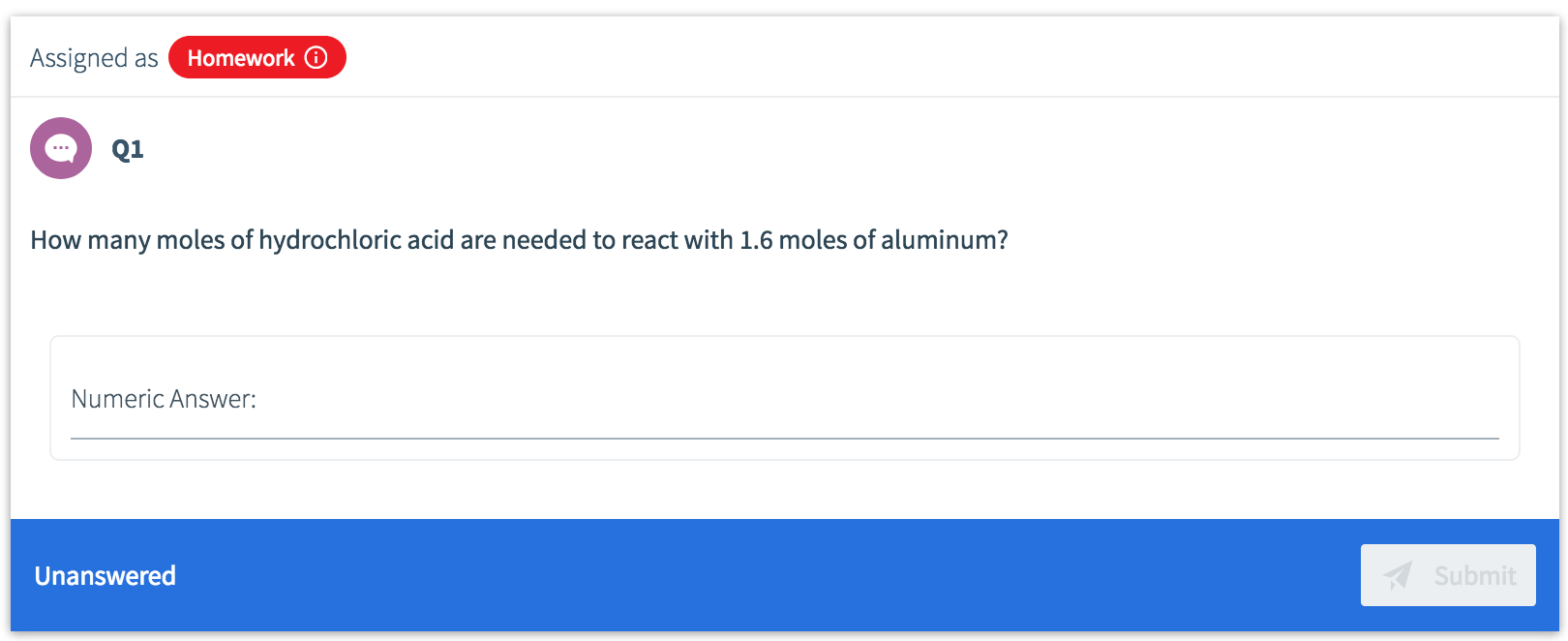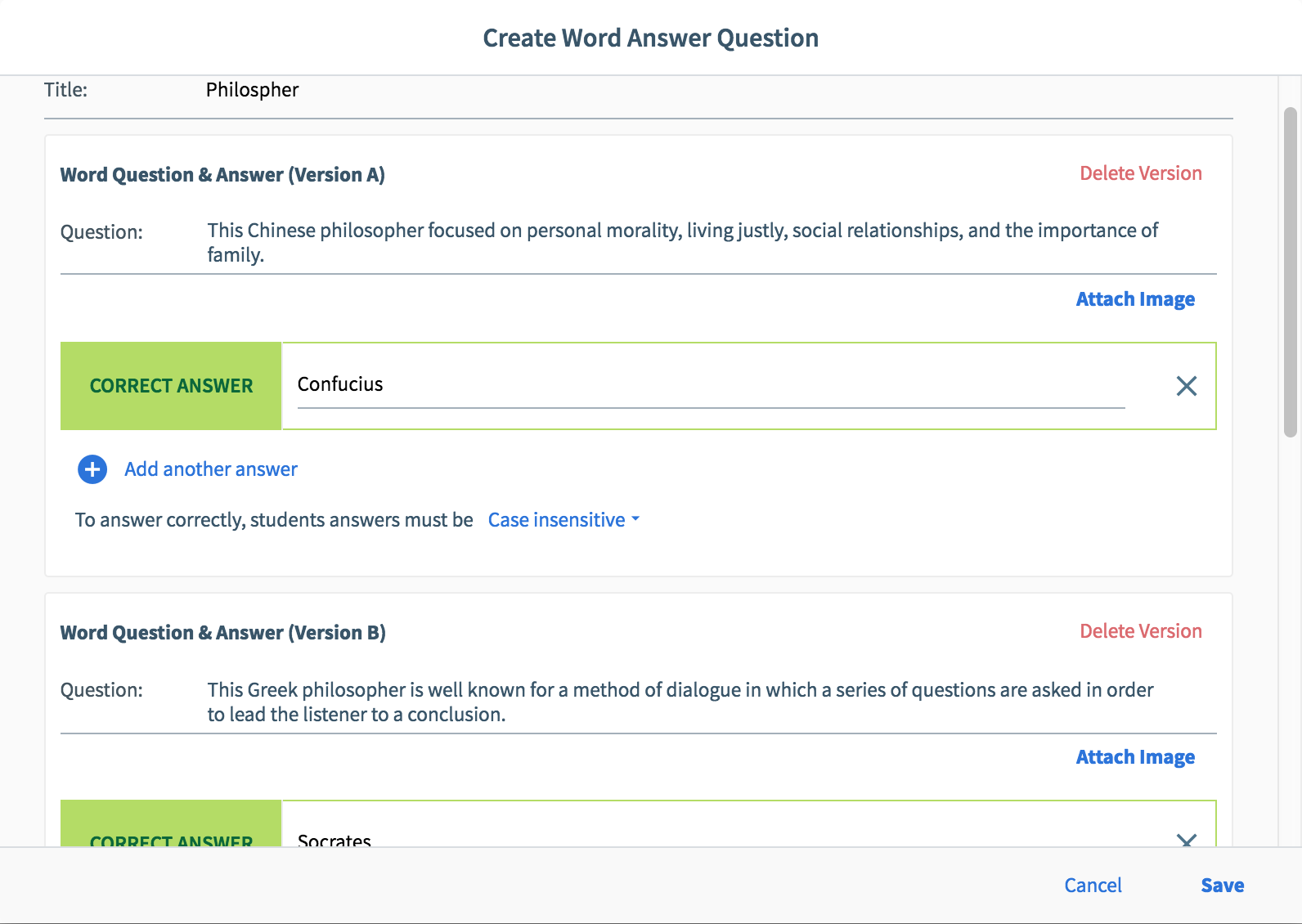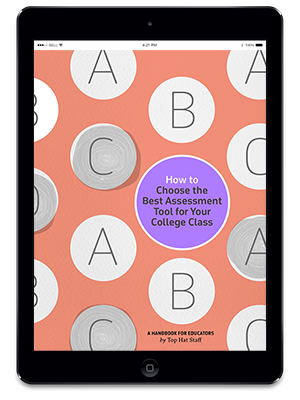Enforcing academic integrity can be a challenge when it comes to setting assignment or test questions. One way Top Hat can help is through randomly generated questions, where you can assign a numerical range or a set of phrases to choose from—and have them automatically graded.

There are two question types, which can be accessed from Pages and Top Hat Test: multi-version questions and formula questions. These need only to be set up once, and then can produce different questions within a range or from a random selection of questions and answers. This makes it less likely for students to receive the same questions as one another, preventing them from copying each other’s work.

Both question types can be used in tests, along with Top Hat Test’s proprietary lock-out capabilities. They also work very well for at-home assignments where students are encouraged to work together but submit their own responses. To get to the answer, each student must understand the concept—not guess or copy the solution. Here’s a look at how both types of question can function in Top Hat:

1. ### Formula questions

2. Formula questions allow STEM instructors to create a question where a formula must be used to get to the solution. If you plug in the formula and the range for each variable involved, Top Hat does the rest to randomly generate up to 15 different versions of your question. Each of your students randomly receives one of the auto-generated questions.

One higher order activity you can try with students is coming up with a formula to a posed problem together as a class. You can then use the formula you created together in a randomly generated question and assign it to your students to solve.

### Setting up a formula question…### …and how it looks to a student.3. ### Multi-version questions

When creating an assignment or Top Hat Test, you can use the Add another version option in most question types to make up to 25 versions of the same question. Each student will randomly receive one of the question versions.

Unlike formula questions, this is word-based. You don’t have to write 25 separate questions, either—they can be the same question but with only one or two words changed, such as a definition.

Multi-version questions can be used in a variety of ways. You can simply change the order in which the answer options appear or you can make different questions that test students on the same concept.Read more about how to use Top Hat’s multi-version and formula question types in the Top Hat Success Center, our comprehensive knowledge base: